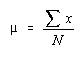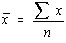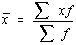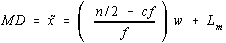Measures of Central Tendency

The term "Average" is vague

Average could mean one of four things. The arithmetic mean, the median, midrange, or mode. For this reason, it is better to specify which average you're talking about.

Mean

This is what people usually intend when they say "average"

Population Mean:Sample Mean:Frequency Distribution:The mean of a frequency distribution is also the weighted mean.

Median

The data must be ranked (sorted in ascending order) first. The median is the number in the middle.

To find the depth of the median, there are several formulas that could be used, the one that we will use is:
Depth of median = 0.5 * (n + 1)

Another way to find the median is to use the techniques used to find the measures of position. Since the median is the number in the middle, you take 1/2 of the sample size and then either add 0.5 if it is an integer or round up if it's not. Then take the ranked value in that position.

Raw Data

The median is the number in the "depth of the median" position. If the sample size is even, the depth of the median will be a decimal -- you need to find the midpoint between the numbers on either side of the depth of the median.

Ungrouped Frequency Distribution

Find the cumulative frequencies for the data. The first value with a cumulative frequency greater than depth of the median is the median. If the depth of the median is exactly 0.5 more than the cumulative frequency of the previous class, then the median is the midpoint between the two classes.

Grouped Frequency Distribution

This is the tough one.

Since the data is grouped, you have lost all original information. Some textbooks have you simply take the midpoint of the class. This is an over-simplification which isn't the true value (but much easier to do). The correct process is to interpolate.

Find out what proportion of the distance into the median class the median by dividing the sample size by 2, subtracting the cumulative frequency of the previous class, and then dividing all that bay the frequency of the median class.

Multiply this proportion by the class width and add it to the lower boundary of the median class.Mode

The mode is the most frequent data value. There may be no mode if no one value appears more than any other. There may also be two modes (bimodal), three modes (trimodal), or more than three modes (multi-modal).

For grouped frequency distributions, the modal class is the class with the largest frequency.

Midrange

The midrange is simply the midpoint between the highest and lowest values.

Summary

The Mean is used in computing other statistics (such as the variance) and does not exist for open ended grouped frequency distributions (1). It is often not appropriate for skewed distributions such as salary information.

The Median is the center number and is good for skewed distributions because it is resistant to change.

The Mode is used to describe the most typical case. The mode can be used with nominal data whereas the others can't. The mode may or may not exist and there may be more than one value for the mode (2).

The Midrange is not used very often. It is a very rough estimate of the average and is greatly affected by extreme values (even more so than the mean).
 Property Mean Median Mode Midrange Always Exists No (1) Yes No (2) Yes Uses all data values Yes No No No Affected by extreme values Yes No No Yes

Using the TI-82

One can find the mean, median, and midrange using the list functions of the TI-82. However, an easier way is to use the one-variable statistics. It will give the mean and median as well as the information necessary to find the midrange. You can also find the measures of variation with the TI-82 calculator.